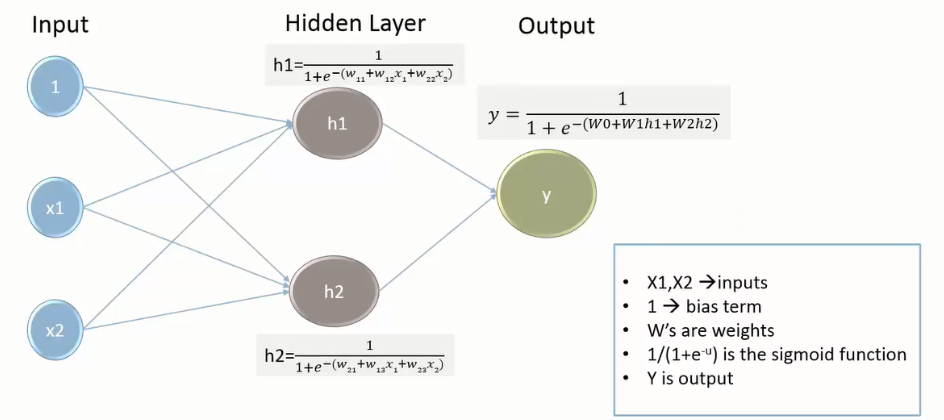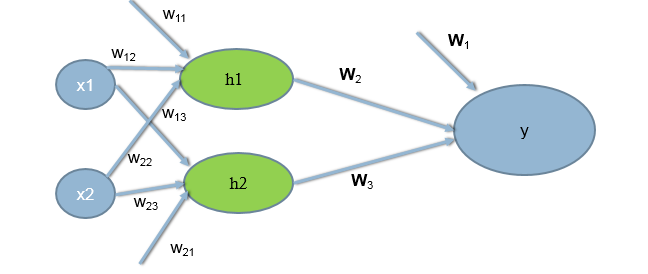• No products in the cart.

# 203.5.6 Neural Network Intuition

##### Neural network at a glance.

In previous section, we studied about  Implementing Intermediate outputs in R

Before going further into neural network algorithm, we need to understand and break down how the algorithm is working.

### Neural Network Intuition

Final Output

(y = out(h) = g(sum W_j h_j))

(h_j = out(x) = g(sum w_(jk)x_k))

(y = out(h) = g(sum W_j g(sum w_(jk) x_k)))

• So h is a non linear function of linear combination of inputs – A multiple logistic regression line
• Y is a non linear function of linear combination of outputs of logistic regressions
• Y is a non linear function of linear combination of non linear functions of linear combination of inputs

We find W to minimize $$\sum_{i=1}^n [y_i – g(\sum W_j h_j)]^2$$ We find $${W_j}$$ and $${w_(jk)}$$ to minimize $$\sum_{i=1}^n [y_i – g(\sum W_j g(\sum w_(jk) x_k))]^2$$ Neural networks is all about finding the sets of weights $${W_j}$$ and $${w_(jk)}$$ using Gradient Descent Method.

### The Neural Networks

• The neural networks methodology is similar to the intermediate output method explained above.
• But we will not manually subset the data to crate the different models.
• The neural network technique automatically takes care of all the intermediate outputs using hidden layers
• It works very well for the data with non-linear decision boundaries
• The intermediate output layer in the network is known as hidden layer
• In Simple terms, neural networks are multi layer nonlinear regression model.
• If we have sufficient number of hidden layers, then we can estimate any complex non-linear function

#### Neural Network and VocabularyWhy are they called hidden layers?

• A hidden layer “hides” the desired output.
• Instead of predicting the actual output using a single model, build multiple models to predict intermediate output.
• There is no standard way of deciding the number of hidden layers.

#### Algorithm for Finding Weights• Algorithm is all about finding the weights/coefficients
• We randomly initialize some weights; Calculate the output by supplying training input; If there is an error the weights are adjusted to reduce this error.

The next post is The Neural Network Algorithm.

21st June 2017

### 0 responses on "203.5.6 Neural Network Intuition"

Statinfer Software Solutions LLP

Software Technology Parks of India,
NH16, Krishna Nagar, Benz Circle,